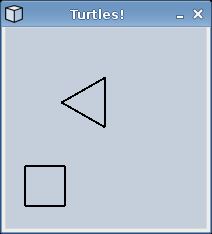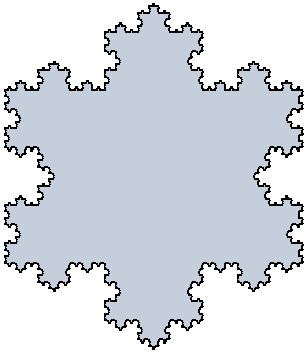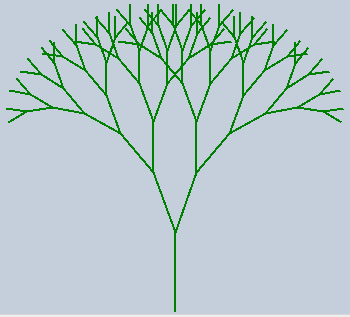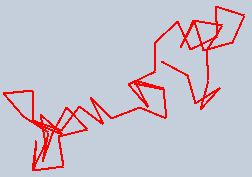# Lab 10: Turtles and Fractals

Due 11:59pm Tuesday, April 15, 2008

A skeleton version of the program will appear when you run update21 in a terminal window. The program handin21 will only submit files in this directory.

Some of the problems will have optional components that allow you to further practice your skills in Python. Optional portions will not be graded, but may be interesting for those wanting some extra challenges.

Introduction
For this assignment you will write a program that implements a class of turtle objects. These are no ordinary turtles however as these turtles have a penchant for both the fine arts and computer science. These are turtles that can draw. Imagine placing a turtle on a canvas with a pen attached to his tail. This turtle can move forward and backward, turn right or left, and lift up or put down its tail. If the turtle moves while its tail is down, the pen leaves a trail behind the path of the turtle. You will write a class that allows you to create and manipulate drawing turtles. Then you will use this class to draw fractals.

A Turtle class
The current state of the turtle can be described by the following variables:
• xPos - The horizontal location of the turtle.
• yPos - The vertical location of the turtle.
• direction - The direction the turtle is heading, measured in degrees counter-clockwise from East.
• tailDown - A Boolean flag that is True if the turtle's tail is lowered.

Begin by implementing the constructor and string methods:

• The constructor __init__ should take two parameters indicating the height and width of the turtle graphics window. The constructor should first create a new GraphWin object using the graphics class. The center of the graphics window should be (0,0) meaning that the lower left and upper right corners of the window should have coordinates (-width/2, -height/2) and (width/2, height/2), respectively. You can use the setCoords method on GraphWin objects to set up this coordinate system. The constructor should place the turtle in the center of the window facing East with the turtle's tail down (in drawing mode).
• The string method __str__ should return the status of a turtle object as a string. For example:
```Position: (0, -249)
Direction: 90 degrees
Tail down? True
```

To change or query the turtle's state, you can use a number of Turtle methods:

The following methods operate on the Turtle's position. Remember that when the turtle's tail is down, it should draw a line in the window as it moves.
• forward(dist) - Move the turtle forward by the given dist in the direction the turtle is currently heading. To do this you will need to move the turtle from its current location (x1,y1) to a new location (x2, y2). If the current turtle heading is dir, then the location of (x2, y2) is given by the following equations:
```x2 = x1 + dist*cos(dir)
y2 = y1 + dist*sin(dir)
```
where dir is measured in radians. To convert from degrees to radians, use the radians function in the math library.
• goto(x,y) - Move (in a straight line) to the location specified by the point (x, y).
• position() - Return the (x,y) location of the turtle as a tuple.

These methods operate on the Turtle's direction:
• right(angle) - Turn angle degrees to the right (clockwise). If the turtle is facing North, then right(90) would make the turtle face East while right(270) from North would make the turtle face West.
• left(angle) - Turn angle degrees to the left (counter-clockwise). If the turtle is facing North, then left(90) would make the turtle face West while left(270) from North would make the turtle face East.

These methods operate on the Turtle's pen:
• up() - Raise the turtle's tail and stop drawing.
• down() - Lower the turtle's tail and start drawing.

These methods operate on the Turtle's graphics window:
• window_height() - Return the height of the graphics window.
• window_width() - Return the width of the graphics window.
• done() - Done drawing - wait for a mouse click and close the window.

Testing the Turtle class
Turtle objects exist in a graphics window, whose center has the coordinates (0,0). When the turtle moves with its tail lowered, a line is drawn in the graphics window that tracks the turtle's movement. An example drawing is shown below for a turtle that initially starts facing East in the center of a 200x200 window.Below is the code that was used to create this image:
```  trevor = Turtle(200, 200)  # at (0,0) facing East
trevor.left(90) # turn left 90 degrees (now facing North)

# A triangle
for i in range(3):
trevor.forward(50)  # move forward 50 steps
trevor.left(120)    # turn left 120 degrees

trevor.up()           #stop drawing
trevor.goto(-80,-80)  #go to (-80, 80)
trevor.down()         #resume drawing

# A square
for i in range(4):
trevor.forward(40)  # move forward 40 steps
trevor.right(90)    # turn right 90 degrees

trevor.done()         # wait for mouse click to close window
```

You can also test your Turtle class with the drawHouse function that is included in the turtle.py file. Be sure to test the Turtle class on these examples before implementing the fractals described below.

Koch curves
The textbook gives an example on page 465-466 of a fractal called the "Koch curve" that can be drawn recursively. You will write a function koch(turtle, length, degree) which draws a Koch curve of length length and degree degree. The koch function is not a method of the Turtle class.

The Koch curve is recursively defined:

• A Koch curve of degree 0 is a line of the specified length.
• A Koch curve of degree n is formed by four Koch curves as follows:
1. drawing a Koch curve of length length/3 with degree n-1
2. turning 60 degrees to the left
3. drawing a Koch curve of length length/3 with degree n-1
4. turning 120 degrees to the right
5. drawing a Koch curve of length length/3 with degree n-1
6. turning 60 degrees to the left
7. drawing a Koch curve of length length/3 with degree n-1

Koch snowflakes

Write a function snowflake(turtle, sides, length, degree) that can draw a Koch snowflake, as described on page 465-466. A Koch snowflake is formed by putting together multiple copies of the Koch curve. The snowflake function is not a method of the Turtle class.

The algorithm for drawing a Koch snowflake with n sides is:

```for each side:
draw a Koch curve of the appropriate length and degree
turn 360/n degrees
```
The snowflake shown below is an example of a Koch snowflake made with 3 Koch curves.c-Curve Fractal

Write a function cCurve(turtle, length, degree) which draws a c-Curve, as described on page 466-467. See the text for an example picture. The cCurve function is not a method of the Turtle class.

C-curves are defined recursively:

• A c-Curve of degree 0 is a line of length length.
• A c-Curve of degree n is formed as follows:
1. turning 45 degrees to the left
2. drawing a c-Curve of length length / sqrt(2) with degree n-1
3. turning 90 degrees to the right
4. drawing a c-Curve of length length / sqrt(2) with degree n-1
5. turning 45 degrees to the left

Optional Components
You may include any additional methods in your class. Some of these methods can make writing other methods easier or extend the feature of you class. As noted above, these questions are NOT required to receive full credit. Furthmore, do not attempt to solve these problems until the required portion of the assignment is complete. There are many extensions you could add to this program. Here are a few we thought might be interesting.
• Add a method backward(dist) which moves the turtle backward dist steps in the direction the turtle is currently facing.
• Add a method color(name) which sets the pen color using the color name specified. The default color is "black".
• Add a method width(w) which sets the thickness of the line drawn by the turtle to w.
• Add a method setHeading(angle) which sets the orientation of the turtle to angle.
• Add a method tracer(flag) which toggles whether or not a "turtle" is drawn on the screen showing its position and orientation. Rotating the turtle can be rather tricky, but if you use methods similar to the rotateBug and animateBug from homework 4, it can be done. Your turtle could be something simple like an filled arrow.
• Recursive techniques can be used to draw trees, ferns, and other patterns. The tree below is drawn by drawing the trunk, turning slightly to the left and recursively drawing a tree, then turning slightly right and recursively drawing a tree. Experiment with recursive drawing and come up with your own patterns.• Simulate a random walk by having the turtle repeatedly turning in a random direction and moving a small distance. An example random walk may look like this:Submit

Once you are satisfied with your program, hand it in by typing handin21 in a terminal window.